# Optimization of the Stability and Reliability of Rotor System by the Methodology of Design Experiments

Optimization of the Stability and Reliability of Rotor System by the Methodology of Design Experiments

LMSS Laboratory, Department of Mechanical Engineering, University of Djillali Liabes, Sidi Bel Abbes 22000, Algeria

Department of Electrical Engineering, University of Djillali Liabes, Sidi Bel Abbes 22000, Algeria

Laboratory of Materials and Reactive Systems, LMSR, Djillali Liabes University, Sidi Bel Abbes 22000, Algeria

Gas Turbine Joint Research Team, Faculty of Science and Technology, University of Djelfa, Djelfa 17000, Algeria

Corresponding Author Email:
mostefa.brihmat@univ-sba.dz
Page:
302-314
|
DOI:
https://doi.org/10.18280/mmep.080219
1 October 2020
|
Revised:
3 January 2021
|
Accepted:
15 January 2021
|
Available online:
28 April 2021
| Citation

OPEN ACCESS

Abstract:

The dynamic behavior of the rotors is influenced by the bearings mounted on them. The aim of this research is to study the effect of the stiffness, shaft diameters and dimensions of lubricated journal bearings on the stability of the system using DOE methodology. We first calculated the values of the real frequency and imaginary frequency of the rotor by the Matlab program, then we prepared a matrix containing forty eight tests according to the Placket Berman plan representing the number of experiments and nine columns representing the factors: the rotor diameters and the dimensions of the hydrodynamic bearing and the system stiffness, to find out the most factors affecting the frequency in The real part or the fictional part, and any of them, affects system stability. The results showed that the diameter D1 has the more significant positive effect on the real part frequency, compared to outer journal diameter Db that has a negative effect on the imaginary part frequency and vice versa, as affirmed by the plot of principal effects. By with this criterion one can estimate the frequency as well as the mode for which the system will become unstable. On the other hand, a finite element code has been written in MATLAB to know the eigenvalues, and critical velocity that correspond to the speed at which the unbalanced excitation coincides with the eigenvalue. The results of the tests showed that the finite element method (FEM) was very effective studying system stability.

Keywords:

Plakett-Burman, hydrodynamic bearings, stability, stiffness, gyroscopic forces, critical rotational speeds

1. Introduction

The development of efficient and accurate numerical procedures to analyze the dynamic phenomena involved on rotor-bearing systems have been motivated mainly by the analysis, design and commissioning of high-speed rotating machinery [1, 2]. In the modeling of rotating machines, some of the main features of the system should be considered, such as gyroscopic effects, rotatory inertia and the bearing contribution. When establishing the stiffness and damping coefficients associated with hydrodynamic rotating bearings in the rotor model, it may be demonstrated that they play an important role in the rotor response.

Statistical experimental design, also known as design of experiments (DOE), is the methodology of how to conduct and plan experiments in order to extract the maximum amount of information with the lowest number of analyses . A désignes experiment is a tool or set of tools used for gathering test data. Typical characteristics of an experimental design are planned testing, data analysis approach, simultaneous factor variability and scientific approach . Various industrial applications relate to rotating machines, such as gas turbines, turbojets, turbochargers, and others. The rotor is the main element which generates vibrations in all rotating machines, particularly during their operations, these vibrations are the results of an unbalance fault .

A rotor can be defined as a combination of shaft and multiple discs suspended on rigid or flexible bearings that allow it to rotate freely about a fixed axis. The discs are often considered to be rigid and the shaft, flexible. The calculation and subsequent control of unbalance response amplitude and stability limit speed have always been of utmost importance in all areas related to turbo machinery. This is especially true in the case of high-speed machines where uncontrolled vibration and instability of rotating machinery can lead to catastrophic results. Another crucial demand in modern designs is the need for a higher power-to-weight ratio. Delivering better performance with lighter machines has added advantages of easier maintenance and transport as well. Considering all such requirements, rotors are designed to be lighter, more flexible, and easily operable at higher speeds.

Earliest among the studies on optimization techniques applied in the field of rotor dynamics has been minimizing a single-objective function of the response due to system unbalance. Pilkey et al.  employed a more efficient linear programming model as opposed to trial-and-error methods that were prevalent before. Bhat et al.  investigated the influence of journal bearing parameters like bearing diameter, clearance, and oil viscosity in optimum rotor design by minimizing unbalance response amplitude in the operating speed range by using the method of feasible directions. Stocki et al.  minimized the vibration amplitude of a compressor shaft while subject to multiple practical constraints on displacements that caused rubbing effects. Helfrich et al.  used optimization techniques to maximize the first critical speed of a multidisc rotor system with bearing and disc dimensions as the design variables. Yucel et al.  optimized unbalance response amplitude of a rotor-bearing system experimentally using the Taguchi method to determine the design variables having the greatest influence on the objective function. While the system used in Pilkey et al.  and Bhat et al.  do not account for mass of the shaft or gyroscopic effects, all the studies discussed above consider optimization of a single-objective function.

Therefore, it’s essential to reduce vibrations to ensure safe and stable operation of considered machine. This can be realized by proper investigation of the system dynamics. The modal analysis is carried out to get an idea of the dynamic behavior of the system.

A very basic model of a rotor was provided by Jeffcott . First, he considered three hypotheses which are: (i) the rotor carries a point mass, (ii) axially symmetrical rotor, and (iii) no damping is related to the rotor. Then, the model was extended to take damping into account. Irretier et al.  constructed a mathematical formulation for the modal analysis of the entire shaft-rotor system at first as a linear time independent system (LTI) and later as a Linear Time Varying system (LTV). Rotor rotation generates additional forces such as gyroscopic, tangential, and rotating damping forces . Because of the effects of these forces, the structure of system matrices becomes asymmetric and depends strongly on the speed .

The stability analysis of symmetrical rotor bearing systems was studied by Laszlo  using finite element method, he taken in consideration the internal damping. His findings showed that the whirling motion of the rotor system becomes unstable beyond the critical speed of instability; he found that the rotor stability is enhanced by increasing of bearings damping.

Fegade et al.  and Patel et al.  in their works, thy studied the harmonic analysis of the rotor to identify the frequency using the variation of the diameter by design optimization (DOE) and by parametric design using ANSYS software. In addition, another study was carried out to develop an alternative procedure called harmonic analysis to identify the frequency of a system through critical speed, amplitude, and phase angle curves using ANSYS.

A study was performed using the Plakett-Burman statistical method on the experimental designs in order to define the influence of the stiffness coefficients on the rotating machines dynamics in particular on the diameters which produce these high frequencies . The factors interactions can affect by increasing or decreasing the principal effects as affirmed by the interaction and surface graphs. Their results show that the inclusion of the stiffness coefficients on the dynamic analysis of rotating machines supported on hydrodynamic bearings play a significant role on the estimation of the unbalance response of rotors.

Naouri Abdallah et al. . In their study, they considered that the dynamic behavior of fluid film bearings is one of the main factors, which affects the rotating machine performances. In this study, a rigid rotor supported by two identical hydrodynamic bearings is taken into consideration. The principal goal of this work is to predict the effect of the damping film of the hydrodynamic bearings on the rotating machines stability.

This paper Concerns the optimization and the modeling of the stability and reliability of operation of a rotor system using the methodology of design of experiments (DOE).

First, we calculated the values of real frequency and imaginary frequency of the rotor by the Matlab program then set up a matrix containing forty eight tests according to the Placket Berman plan which represents the number of experiments and nine columns representing the factors, which are the following the diameters of the rotor, and the dimensions of the hydrodynamic bearing, and the rigidity of the system, in order to know the most influencing factors on the frequency in the real part or the imaginary part, and which of them have an impact on the destabilization of the system. It was found by the results that the factors having a positive effect on the increase in frequency in the real part have a negative effect on the frequency in the imaginary part. Finite element code is written in MATLAB to determine the eigenvalues and eigen vectors. Eigenvalues are calculated in imaginary parts, that show the system natural frequencies, which are used to plot the Campbell diagram. The stability diagram is plotted using the maximum real fraction of all eigenvalues with rotational velocity. Eigenvalues are also used to study the effect of a typical damping factor on rotational speed. These diagrams show that the stability of the system can be studied and the rotational speed stability limit can be determined.

2. Geometric Characteristics

Figure 1 illustrates the cross section of a "plain" journal bearing . The bearing main dimensions are: $D_{B}=2 R_{B}$ - inner bearing diameter, $D=2 R$ -outer journal diameter, $L$ - bearing length, $C=R_{B}-R$ - bearing clearance, $e=\overline{o_{B} o_{J}}-$ eccentricity, $\phi$ - attitude angle and $h$ - fluid film thickness.

## 1.png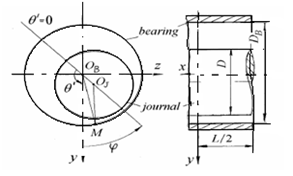Figure 1. Geometry of a plain cylindrical bearing

It is necessary to present the following dimensionless engineering parameters: $L / D$ - length-to-diameter ratio; $C / R$ - clearance ratio; $\varepsilon=e C-$ eccentricity ratio; and $\bar{h}==h / C-$ normalized film thickness.

The journal rotates anticlockwise with a constant angular speed $\Omega=2 \pi N(\mathrm{rad} / \mathrm{sec})$, where $N$ represents the journal spin speed in rps.

3. Constitutive Relations and Equations of Motion

3.1 Effects of the bearings

The bearings act as external forces acting on the rotor. They are characterized by their stiffness and damping.

$F_{u}=-K_{x x} u-k_{x z} w-C_{x x} \dot{u}-C_{x z} \dot{w}$

$F_{w}=-K_{z z} w-k_{z x} u-C_{z z} \dot{w}-C_{z x} \dot{u}$     (1)

In matrix form:

$[F]=-[K] \delta-[C] \ddot{\delta}$     (2)

With stiffness            With bearing damping

$[K]=\left[\begin{array}{cccc}k_{x x} & 0 & k_{x z} & 0 \\ 0 & 0 & 0 & 0 \\ k_{z x} & 0 & k_{z z} & 0 \\ 0 & 0 & 0 & 0\end{array}\right]\left[C_{d}\right]=\Omega\left[\begin{array}{cccc}0 & 0 & 0 & 0 \\ 0 & 0 & 0 & 0 \\ 0 & 0 & 0 & -I_{D y} \\ 0 & 0 & I_{D y} & 0\end{array}\right]$     (3)

3.2 Equations of the rotor movement

The application of the Lagrange equations on the different energies gives:

For the shaft:

$\frac{d}{d t}\left(\frac{\partial T}{\partial \dot{\delta}}\right)-\frac{\partial T}{\partial \delta}=\left(M+M_{S}\right) \ddot{\delta}+C \dot{\delta}$     (4)

With mass and gyroscopic effect matrices. The mass matrix is symmetrical. The matrix C is antisymmetric.

$\frac{\partial U}{\partial \delta}=\left(k_{c}+k_{F}\right) \delta$    (5)

Kc takes into account the effect of shear, KF is due to axial forces.

For the disc:

$\frac{d}{d t}\left(\frac{\partial t}{\partial \dot{\delta}}\right)-\frac{\partial T}{\partial \delta}=M_{d} \ddot{\delta}+C_{d} \dot{\delta}$     (6)

$\left[M_{d}\right]=\left[\begin{array}{cccc}k_{x x} & 0 & 0 & 0 \\ 0 & M_{D} & 0 & 0 \\ k_{z x} & 0 & I_{D x} & 0 \\ 0 & 0 & 0 & I_{D z}\end{array}\right][C]=\left[\begin{array}{cccc}c_{x x} & 0 & c_{x z} & 0 \\ 0 & 0 & 0 & 0 \\ c_{z x} & 0 & c_{z z} & 0 \\ 0 & 0 & 0 & 0\end{array}\right]$     (7)

From equations of the shaft, the disk and the bearings the equation of the rotor motion is written in the form:

$M \ddot{\delta}+C(\Omega) \delta+\dot{K} \delta=0$     (8)

The mass matrix contains the mass of the rotor and disks. The stiffness matrix contains the stiffness of the shaft and bearings. C contains the gyroscopic effect of the shaft and the disks and the matrix damping of the bearings.

The assembly of the displacement vectors of all the rotor nodes into finite elements gives the global displacement vector {X} and the global matrices. In this case the equation of rotor motion becomes:

$[M]\{\ddot{X}\}+\left(\Omega\left[C_{g}\right]+\left[C_{p}\right]\right)\{\dot{X}\}+\left(\left[K_{r}\right]+\left[K_{p}\right]\right)\{X\}=0$     (9)

The gyroscopic effect of the shaft and disks and the matrix damping of the bearings. F can represent unbalance or any other external forces.

3.3 Some important phenomena in rotor dynamics

We will now consider some important phenomena of rotor dynamics, and see in particular the concepts of critical velocities and instability related to rotational damping and the role that differences in rotor dynamics play.

• Critical speeds

The critical velocity corresponds with the speed at which the unbalanced excitation coincides with one of the natural frequencies of the system. In machines consisting of organs with significant moments of polar inertia, we observe a strong dependence on eigen patterns versus rotational velocity due to gyroscopic effects. Thus, we observe the duality of the eigen patterns of the system (in the case of the axial symmetry system) due to the gyroscopic forces as follows:

- (FW) as the rotor rotates in the same direction as it rotates. Then, under gyroscopic effects, the associated resonant frequency increases and this is called "direct precession".

- (BW), where the rotor rotates in the opposite direction to its preliminary motion, resulting in a softening effect and thus a decrease in the critical velocity and this is called the "retrograde precession".

• Instability due to rotating damping

In the study of stability of rotating machines where damping corresponds to one of the determinants of sizing systems, damping can be responsible for unstable phenomena at high speed, which may lead to rupture of rotor components. In order to ensure system integrity, the damping ratios on both fixed and moving parts must be estimated.

• Stability analysis

Stability analysis in the study of the vibrational and dynamic behavior of a flexible rotor is essential because it is considered a dynamic system governed by systems of differential equation. The definition of stability covers Leibunov's definition of equilibrium stability analysis and the Poincaré definition of the concept of orbital stability .

We can know the limits of dynamic system instability and in particular in rotor dynamics through the following techniques:

• Sign of the real part of the complex eigenvalues of the equation system in free motion. If the eigenvalues is given by s = -a ± jb, the only instability is determined when a becomes negative (real positive part), with this criterion one can estimate the frequency as well as the mode for which the system will become unstable. The Routh-Hrwitz criterion makes it possible to analyze the stability of autonomous systems .

The use of this criterion is interesting for systems with a few degrees of freedom, since the analytical expressions of the characteristic polynomial associated with the perturbed motion can be inferred. However, it becomes complicated for systems that include a large number of degrees of freedom. Additionally, this criterion does not provide an instability frequency.

These two criteria studying the stability of a dynamic system restrict particular cases, or when they are described by linear models, for example R. Sino . In the subject of his thesis, he uses these two methods to study and analyze the stability of a rotor due to rotating damping. A more general method is based on Floquet's theory.

• Floquet's theory allows us to analyze, in the linear sense, the stability of systems with periodic coefficients . The first step of analysis consists in applying a vector of the periodic solution S p to a small disturbance δ s. This brings the system to a new state of equilibrium. The second, we apply a first order Taylor expansion to the neighborhood of S p to estimate the nonlinear forces in (s p + δ s, ṡ p + δ ∙ s, s ∙∙ + δ ∙∙ s) in order to make the system linear. This technique is frequently used in the dynamic analysis of rotating structures, particularly in the dynamics of nonlinear rotors (see, for example, the work of Dugundji and Wendell . On the stability analysis of a wind turbine and the analysis of the stabilities of a cracked rotor by Meng and Gasch ).
4. Methodology

The main objective of this work is to model and optimize the operation of the rotor system using DOE methodology and to know the gyroscopic effect on the subjective values of the rotor-bearing systems through the Campbell scheme, and to calculate the imbalance responses mainly during the passage of critical velocity. In the use of finite element method. We designed a mathematical model under the rotor kit name in the matlab program that contains the engineering data for the rotor group element (tree data, disk data, and engineering data for the rotor group component.

In addition to the stiffness and damping matrices in the form of a group of nodes and elements to calculate the values of hardness and the imaginary and real frequency in the presence of speed 0-10000 rpm. This calculation mode should be able to give the geometry of the rotor in finite elements. The search for eigenvalues is an essential process in the study of rotor dynamics.

## 2.png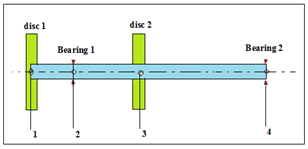Figure 2. Schematic model of the used model of rotor kit

• Rotor Kit

The used model is a rotor kit with a length of 0.42m as shown in Figure 2. A mass of 1.415 kg is mounted on the shaft which is supported by two bearings respectively 0.09 (m) and 0.42 (m) apart from the left end. four stations are considered during harmonic analysis as shown in Figure 1, where station numbers denote different nodes in the model (1) Disc, (2) First bearing node, (3) Disk, (4) Second bearing. For the distributed rotor and the concentrated disc (1), the material density is 7850 kg / m3 and the modulus of elasticity is 2.06E11 N / m2. with a mass of 0.81 kg, disk (4) with a mass of 0.6050 kg polar inertia (5.783e-4, 0 ,4.320e-4) kg.m2 and diametral inertia (3.357e-4,0, 2.433e-4) kg.m2.

Calculation of real and imaginary system frequency values

A mathematical model was designed under the name of rotor kit using Matlab which includes the data of each element of the rotor kit (shaft, disc, and bearing), and also the stiffness and damping matrices in the form of a set of nodes and elements were used to determine the values of the stiffness and the real and imaginary frequency at a speed interval ranging from 0 to 10,000 rpm (Table 1).

Table 1. Geometric data of rotor-bearing element

 Element Node No Node Location (cm) Bearing and Disk Inner Diameter (cm) Outer Diameter (cm) 1 0 disc 0 0.009525 2 0.09 Bearing 0 0.009525 3 0.22 disc 0 0.009525 4 0.42 Bearing 0 0.009525
5. Results and Discussion

5.1 Response

The response chosen is the real frequency and the imaginary frequency of a rotor system - bearings, calculated by matlab software.

5.2 Determination of factors and field of study

The factors examined in this study are:

- The diameters of the rotor kit d1, d2, d3.d4.

- The dimensions of the hydrodynamic bearings

Lb = length of bearings [m].

Db = outer journal diameter [m].

Cb = bearing cleareance [m].

- The shaft is mounted on two fluid film bearings where the stiffness (Kyy, Kzz) was determined using Matlab software.

- Kyz= Kyz = 0.

- The components of damping are taken as: Czz = Cyy = 61, 4000 (Ns / m). The imbalance response for a counted disc center eccentricity of 0.635 (cm) at station (2) was estimated for a speed interval of 0 to 10000 rpm.

5.3 Choice of the experimental design

First, a screening plan is used. This is a first degree plan which allows you to sort the factors by highlighting the most influential. We chose the Plackett-Burman plan because it is generally the most used in similar studies due to its economy in terms of number of tests.

The nine factors studied and their field of study were grouped together in Table 2.

Table 2. Caption

 Levels Units Symbol Factors +1 -1 m)) d1=d2=d3=d4 The diameters 0.050 0.10 0.00009 0.045 0.09 0.00005 )m) (m) m)) Lb Db Cb The dimensions of the hydrodynamic bearings 2,2e5 0 Ns/ m Kyy= Kzz Stiffness
6. Plackett-Burman Screening Plan

6.1 Carrying out the tests

The tests are conducted according to the Plackett-Burman plan for the 9 factors. The experiments took place according to the matrix of this plan. It represents the fixing of factors at different levels, as shown in Table 3.

Table 3. Plackett-Burman plan based on the experimental matrix

 d1 d2 d3 d4 Lb Cb Db Kyy Kzz FRQ img FRQ real 1 0.009475 0.009475 0.009575 0.009475 0.045 0.1 0.00009 2.2E+05 0.0E+00 16.463 310.192 2 0.009575 0.009475 0.009575 0.009575 0.05 0.1 0.00009 0.0E+00 0.0E+00 44.0043 311.976 3 0.009575 0.009575 0.009575 0.009575 0.045 0.1 0.00009 2.2E+05 2.2E+05 145.212 14.0174 4 0.009575 0.009475 0.009575 0.009475 0.045 0.09 0.00009 2.2E+05 0.0E+00 16.463 310.263 5 0.009575 0.009475 0.009475 0.009575 0.05 0.1 0.00009 0.0E+00 2.2E+05 16.617 279.268 6 0.009475 0.009475 0.009475 0.009575 0.05 0.09 0.00009 2.2E+05 0.0E+00 16.616 323.984 7 0.009575 0.009475 0.009475 0.009475 0.045 0.1 0.00005 0.0E+00 0.0E+00 44.5628 325.573 8 0.009475 0.009575 0.009475 0.009475 0.045 0.09 0.00009 2.2E+05 0.0E+00 16.395 324.338 9 0.009575 0.009575 0.009575 0.009475 0.045 0.09 0.00005 2.2E+05 0.0E+00 16.234 310.591 10 0.009475 0.009475 0.009575 0.009575 0.05 0.1 0.00005 2.2E+05 2.2E+05 142.206 13.7369 11 0.009475 0.009475 0.009475 0.009475 0.05 0.09 0.00005 0.0E+00 0.0E+00 44.5475 325.565 12 0.009475 0.009575 0.009475 0.009575 0.045 0.09 0.00005 2.2E+05 2.2E+05 142.17 13.5302 13 0.009475 0.009575 0.009575 0.009475 0.05 0.1 0.00005 0.0E+00 2.2E+05 16.236 310.516 14 0.009475 0.009575 0.009475 0.009575 0.045 0.09 0.00009 2.2E+05 2.2E+05 142.17 13.5302 15 0.009475 0.009475 0.009575 0.009575 0.05 0.09 0.00009 0.0E+00 2.2E+05 16.463 310.192 16 0.009575 0.009475 0.009475 0.009475 0.045 0.1 0.00009 0.0E+00 2.2E+05 16.617 324.052 17 0.009575 0.009575 0.009575 0.009575 0.045 0.09 0.00005 0.0E+00 0.0E+00 16.234 310.591 18 0.009575 0.009575 0.009475 0.009575 0.05 0.09 0.00005 2.2E+05 0.0E+00 16.396 324.409 19 0.009575 0.009575 0.009575 0.009575 0.05 0.09 0.00005 0.0E+00 0.0E+00 44.4545 312.228 20 0.009475 0.009475 0.009575 0.009575 0.045 0.1 0.00005 2.2E+05 0.0E+00 16.463 310.192 21 0.009475 0.009575 0.009475 0.009475 0.05 0.1 0.00009 0.0E+00 2.2E+05 16.395 324.338 22 0.009475 0.009475 0.009475 0.009475 0.05 0.1 0.00005 2.2E+05 0.0E+00 16.616 323.984 23 0.009575 0.009475 0.009475 0.009575 0.05 0.1 0.00005 2.2E+05 0.0E+00 16.617 324.052 24 0.009475 0.009475 0.009575 0.009475 0.045 0.09 0.00005 2.2E+05 2.2E+05 142.206 13.7369 25 0.009475 0.009575 0.009475 0.009475 0.045 0.1 0.00009 0.0E+00 2.2E+05 16.395 324.338 26 0.009575 0.009475 0.009475 0.009575 0.045 0.09 0.00009 2.2E+05 2.2E+05 144.574 13.9962 27 0.009475 0.009475 0.009475 0.009475 0.045 0.09 0.00005 0.0E+00 0.0E+00 44.5475 325.565 28 0.009575 0.009475 0.009575 0.009475 0.05 0.09 0.00005 2.2E+05 2.2E+05 145.563 14.1185 29 0.009575 0.009575 0.009575 0.009475 0.05 0.09 0.00009 0.0E+00 0.0E+00 44.4545 262.112 30 0.009475 0.009475 0.009575 0.009575 0.045 0.1 0.00009 0.0E+00 0.0E+00 43.9888 311.966 31 0.009475 0.009475 0.009475 0.009575 0.045 0.09 0.00005 0.0E+00 2.2E+05 16.616 323.984 32 0.009475 0.009475 0.009475 0.009575 0.05 0.09 0.00009 0.0E+00 2.2E+05 16.616 323.984 33 0.009575 0.009575 0.009475 0.009575 0.045 0.1 0.00005 0.0E+00 0.0E+00 44.9909 325.855 34 0.009475 0.009575 0.009475 0.009475 0.05 0.1 0.00009 2.2E+05 0.0E+00 16.395 324.338 35 0.009575 0.009575 0.009475 0.009475 0.05 0.09 0.00005 2.2E+05 2.2E+05 144.904 13.8962 36 0.009575 0.009475 0.009575 0.009475 0.05 0.09 0.00005 0.0E+00 2.2E+05 16.463 310.263 37 0.009475 0.009575 0.009575 0.009475 0.045 0.1 0.00005 0.0E+00 2.2E+05 16.395 324.338 38 0.009575 0.009575 0.009475 0.009575 0.05 0.1 0.00009 2.2E+05 0.0E+00 16.396 324.409 39 0.009475 0.009575 0.009575 0.009575 0.045 0.1 0.00005 2.2E+05 0.0E+00 16.236 310.516 40 0.009575 0.009575 0.009475 0.009575 0.045 0.1 0.00005 0.0E+00 2.2E+05 16.396 324.409 41 0.009575 0.009475 0.009475 0.009475 0.05 0.1 0.00005 2.2E+05 2.2E+05 144.574 13.9962 42 0.009475 0.009575 0.009575 0.009475 0.05 0.09 0.00009 0.0E+00 0.0E+00 44.4388 312.217 43 0.009475 0.009575 0.009575 0.009575 0.05 0.09 0.00009 2.2E+05 2.2E+05 142.457 13.6378 44 0.009575 0.009475 0.009575 0.009475 0.045 0.1 0.00009 2.2E+05 2.2E+05 145.563 14.1185 45 0.009475 0.009575 0.009575 0.009575 0.05 0.1 0.00005 0.0E+00 2.2E+05 44.4388 312.217 46 0.009575 0.009575 0.009575 0.009475 0.05 0.1 0.00009 2.2E+05 2.2E+05 145.212 14.0174 47 0.009575 0.009475 0.009575 0.009575 0.045 0.09 0.00009 0.0E+00 0.0E+00 44.0043 311.976 48 0.009575 0.009575 0.009475 0.009475 0.045 0.09 0.00009 0.0E+00 0.0E+00 44.9909 325.855
7. Statistical Analysis of the Results

The processing of the experimental data was carried out by linear regression multiple using the MINITAB17 software.

7.1 Graphic representation of effects

This diagram (Figure 3) makes it possible to extract the most important parameters. Among all the factors studied and at the chosen confidence level (α=0.05), the strong factors (kzz) and (kyy) appear to be very influential factors, kzz, kyy having the most significant positive effect in the imaginary part frequency and affects negatively the frequency in real part.

A. Pareto chart:

## 3.png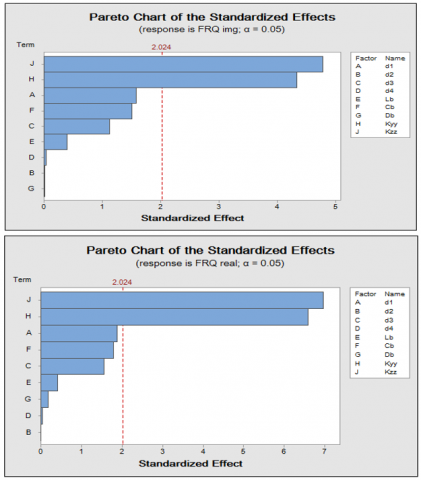Figure 3. Pareto plot of normalized effects

Figure 4 reveals that kzz and kyy has the greatest significant positive effect on the rightmost imaginary frequency of the response line. However, the figure reveals a significant reduction effect of kzz and kyy on the real frequency of its effect is positioned to the left of the answer line.

B. Pareto plot of normalized

## 4.png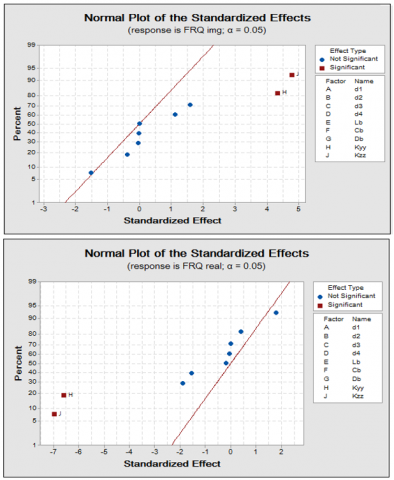Figure 4. Pareto plot of normalized effects

C. Main effects diagram

The main effects diagram tells us about the simultaneous influence of all factors on the frequency. We can from this diagram (Figure 5) conclude that the stiffness kzz and kyy are the most influential factors positively on the imaginary part frequency, and negatively influential on the real part frequency.

## 5.png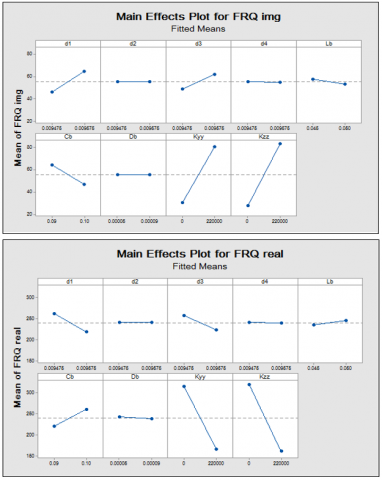Figure 5. Diagram of the main effects on frequency imaginary- real

7.2 Determination of significant effects and coefficients of the model

The effects values and the coefficients of regression of the model are given as bellow in Tables 4 and 5.

Table 4. Factorial Regression: FRQ img versus d1; d2; d3; d4; Lb; Cb; Db; Kyy; Kzz

 Source DF Adj SS Adj MS F-Value P-Value Model 9 71923 7991.5 5.07 0.000 Linear 9 71923 7991.5 5.07 0.000 D1 1 3984 3984.4 2.53 0.120 D2 1 0 0.2 0.00 0.991 D3 1 1986 1985.5 1.26 0.269 D4 1 2 2.2 0.00 0.970 Lb 1 240 239.7 0.15 0.699 Cb 1 3577 3577.1 2.27 0.140 Db 1 0 0.2 0.00 0.992 kyy 1 29663 29662.7 18.80 0.000 kzz 1 36092 36092.3 22.88 0.000 error 38 59946 1577.5 Total 47 131869

Model Summary

S                R-sq          R-sq (adj)          R-sq (pred)

39.7180      54.54%         43.77%              27.61%

Table 5. Factorial Regression: FRQ real versus d1; d2; d3; d4; Lb; Cb; Db; Kyy; Kzz

 Source DF Adj SS Adj MS F-Value P-Value Model 9 595380 66153 10.83 0.000 Linear 9 595380 66153 10.83 0.000 D1 1 21791 21791 3.57 0.067 D2 1 0 0 0.00 0.999 D3 1 14753 14753 2.42 0.128 D4 1 12 12 0.00 0.965 Lb 1 1032 1032 0.17 0.683 Cb 1 19436 19436 3.18 0.082 Db 1 187 187 0.03 0.862 kyy 1 264891 264891 43.38 0.000 kzz 1 296031 296031 48.48 0.000 error 38 232052 6107 Total 47 827431

Summary of model

S             R-sq         R-sq (adj)     R-sq (pred)

78.1449      71.96%        65.31%         55.35%

Table 6. The used Runs in DOE

 d1 d2 d3 d4 Lb Cb Db Kyy Kzz FRQ img FRQ real 1 0.009475 0.009475 0.009475 0.009575 0.045 0.09 0.00009 0.0E+00 2.2E+05 16.616 323.984 2 0.009475 0.009575 0.009575 0.009475 0.05 0.09 0.00009 2.2E+05 0.0E+00 44.439 312.217 3 0.009475 0.009475 0.009575 0.009575 0.045 0.09 0.00005 2.2E+05 2.2E+05 142.46 13.6378 4 0.009575 0.009475 0.009575 0.009475 0.045 0.1 0.00009 2.2E+05 2.2E+05 144.9 13.8962 5 0.009475 0.009475 0.009575 0.009575 0.05 0.1 0.00005 0.0E+00 2.2E+05 44.439 312.217 6 0.009575 0.009475 0.009475 0.009475 0.05 0.1 0.00009 0.0E+00 2.2E+05 16.463 310.263 7 0.009575 0.009575 0.009575 0.009475 0.05 0.1 0.00005 0.0E+00 0.0E+00 44.455 262.112 8 0.009475 0.009575 0.009475 0.009475 0.05 0.1 0.00005 2.2E+05 0.0E+00 16.395 324.338 9 0.009575 0.009575 0.009475 0.009475 0.05 0.09 0.00005 2.2E+05 2.2E+05 145.56 14.1185 10 0.009475 0.009475 0.009475 0.009475 0.05 0.1 0.00005 0.0E+00 2.2E+05 16.395 324.338 11 0.009575 0.009475 0.009475 0.009575 0.045 0.09 0.00005 2.2E+05 0.0E+00 144.57 13.9962 12 0.009475 0.009575 0.009475 0.009475 0.05 0.09 0.00005 0.0E+00 0.0E+00 44.548 325.565 13 0.009475 0.009475 0.009575 0.009475 0.045 0.1 0.00005 2.2E+05 2.2E+05 144.57 13.9962 14 0.009475 0.009575 0.009475 0.009575 0.05 0.09 0.00005 0.0E+00 2.2E+05 44.991 325.855 15 0.009575 0.009575 0.009475 0.009575 0.045 0.09 0.00009 0.0E+00 0.0E+00 16.616 323.984 16 0.009475 0.009575 0.009575 0.009575 0.05 0.09 0.00009 2.2E+05 2.2E+05 44.439 312.217 17 0.009575 0.009575 0.009575 0.009575 0.045 0.1 0.00005 2.2E+05 2.2E+05 142.46 13.6378 18 0.009575 0.009475 0.009575 0.009575 0.045 0.09 0.00005 0.0E+00 2.2E+05 145.56 14.1185 19 0.009575 0.009475 0.009575 0.009575 0.05 0.1 0.00009 0.0E+00 2.2E+05 44.439 312.217 20 0.009575 0.009575 0.009475 0.009575 0.045 0.1 0.00009 2.2E+05 0.0E+00 16.463 310.263 21 0.009475 0.009475 0.009575 0.009575 0.045 0.1 0.00009 2.2E+05 0.0E+00 16.395 324.338 22 0.009575 0.009575 0.009475 0.009575 0.045 0.1 0.00009 0.0E+00 2.2E+05 16.396 324.409 23 0.009575 0.009575 0.009475 0.009575 0.05 0.09 0.00009 2.2E+05 0.0E+00 16.236 310.516 24 0.009475 0.009475 0.009575 0.009575 0.05 0.09 0.00009 2.2E+05 0.0E+00 16.396 324.409 25 0.009475 0.009575 0.009475 0.009575 0.05 0.09 0.00005 0.0E+00 2.2E+05 16.616 323.984 26 0.009475 0.009575 0.009475 0.009475 0.05 0.1 0.00005 0.0E+00 2.2E+05 16.395 324.338 27 0.009575 0.009475 0.009575 0.009475 0.05 0.09 0.00005 2.2E+05 0.0E+00 145.56 14.1185 28 0.009575 0.009575 0.009475 0.009475 0.045 0.1 0.00005 2.2E+05 2.2E+05 144.57 13.9962 29 0.009575 0.009575 0.009575 0.009475 0.045 0.09 0.00009 2.2E+05 2.2E+05 145.21 14.0174 30 0.009575 0.009575 0.009475 0.009575 0.045 0.09 0.00009 0.0E+00 0.0E+00 44.991 325.855 31 0.009575 0.009575 0.009475 0.009475 0.05 0.1 0.00009 2.2E+05 0.0E+00 16.395 324.338 32 0.009575 0.009575 0.009575 0.009475 0.045 0.1 0.00009 0.0E+00 0.0E+00 44.455 262.112 33 0.009475 0.009575 0.009575 0.009575 0.045 0.1 0.00005 0.0E+00 0.0E+00 43.989 311.966 34 0.009575 0.009475 0.009475 0.009475 0.05 0.1 0.00009 0.0E+00 2.2E+05 16.396 324.409 35 0.009475 0.009575 0.009575 0.009475 0.05 0.1 0.00005 0.0E+00 2.2E+05 16.236 310.516 36 0.009575 0.009475 0.009575 0.009475 0.05 0.09 0.00005 2.2E+05 0.0E+00 145.56 14.1185 37 0.009475 0.009575 0.009475 0.009575 0.045 0.1 0.00005 0.0E+00 2.2E+05 43.989 311.966 38 0.009475 0.009475 0.009575 0.009575 0.045 0.09 0.00009 0.0E+00 0.0E+00 16.395 324.338 39 0.009475 0.009475 0.009475 0.009575 0.045 0.1 0.00005 0.0E+00 0.0E+00 16.616 323.984 40 0.009575 0.009575 0.009575 0.009575 0.045 0.1 0.00009 0.0E+00 0.0E+00 16.396 324.409 41 0.009475 0.009475 0.009575 0.009475 0.045 0.1 0.00005 2.2E+05 2.2E+05 142.21 13.7369 42 0.009475 0.009575 0.009575 0.009475 0.05 0.09 0.00009 0.0E+00 2.2E+05 16.395 324.338 43 0.009575 0.009475 0.009475 0.009575 0.045 0.09 0.00005 2.2E+05 0.0E+00 144.57 13.9962 44 0.009475 0.009475 0.009475 0.009475 0.045 0.09 0.00009 0.0E+00 0.0E+00 44.548 325.565 45 0.009575 0.009475 0.009575 0.009475 0.05 0.09 0.00009 2.2E+05 2.2E+05 144.9 13.8962 46 0.009475 0.009475 0.009475 0.009475 0.05 0.09 0.00009 2.2E+05 0.0E+00 16.617 324.052 47 0.009575 0.009475 0.009475 0.009575 0.05 0.1 0.00009 2.2E+05 0.0E+00 16.616 323.984 48 0.009475 0.009475 0.009575 0.009475 0.045 0.09 0.00005 2.2E+05 0.0E+00 142.21 13.7369

7.3 Mathematical model equation

To build the model equation representing the relationship between the frequency (img-real) and the 9 factors studied, we use the regression coefficients shown in Tables 4 and 5. This model has been simplified, and the ranking of factors is done according to the diagram of Pareto (Figures 3 and 4).

FRQ img = -2705 + 182863 d1 – 1239 d2 + 128641 d3 - 4289 d4 – 897 Lb- 1733 Cb + 2952 Db + 0.000226 Kyy + 0.000252 Kzz

FRQ real = 7438 – 427644 d1 – 204 d2 – 350633 d3 – 9860 d4 + 1862 Lb+ 4039 Cb- 98695 Db- 0.000675 Kyy- 0.000721 Kzz

• Optimization method

To enhance the seven remaining response factors, the values are set to P < 0.05, the red reference line was changed to zero by moving manually the columns of the base design matrix, maintaining the corresponding frequency values for each row, and keeping the matrix balanced until building a final matrix as shown in Table 6 .

The goal is therefore to find the optimal polynomial equation. From the previous statistical analysis, eliminating the quadratic terms yields a new well-fitting model. The results are presented in Tables 7 and 8.

Table 7. Factorial Regression: FRQ img versus d1; d2; d3; d4; Lb; Cb; Db; Kyy; Kzz

 Source DF Adj SS Adj MS F-Value P-Value Model 9 142685 15853.9 97.80 0.000 Linear 9 142685 15853.9 97.80 0.000 D1 1 22047 22044.9 135.99 0.000 D2 1 3084 3083.6 19.02 0.000 D3 1 7235 7235.1 44.63 0.000 D4 1 6476 6476.2 39.95 0.000 Lb 1 17393 17393.5 107.30 0.000 Cb 1 15817 15816.9 97.57 0.000 Db 1 32677 32677.4 201.58 0.000 kyy 1 15627 15627.0 96.40 0.000 kzz 1 10429 10429.3 64.34 0.000 error 38 6160 162.1 Total 47 148845
• Model Summary

S             R-sq              R-sq (adj)     R-sq (pred)

12.7320        95.86%            94.88%          93.37%

• Regression Equation in Uncoded Units

FRQ img = -1947 + 461686 d1 - 166044 d2 + 256755 d3 - 255436 d4- 8302 Lb- 3822 Cb- 1379219 Db+ 0.000180 Kyy + 0.000141 Kzz

• Fits and Diagnostics for Unusual Observations

Obs   FRQ img      Fit          Resid         Std Resid

35      16.24        41.07        -24.84          -2.13  R

Table 8. Factorial Regression: FRQ real versus d1; d2; d3; d4; Lb; Cb; Db; Kyy; Kzz

 Source DF Adj SS Adj MS F-Value P-Value Model 9 943547 104839 409.14 0.000 Linear 9 943547 104839 409.14 0.000 D1 1 158263 158263 617.64 0.000 D2 1 27375 27375 106.84 0.000 D3 1 38406 38406 149.88 0.000 D4 1 46773 46773 182.54 0.000 Lb 1 112402 112402 438.66 0.000 Cb 1 75656 75656 295.25 0.000 Db 1 193402 193402 754.77 0.000 kyy 1 123233 123233 480.93 0.000 kzz 1 67846 67846 264.78 0.000 error 38 9737 256 Total 47 104
• Model Summary

S                R-sq       R-sq (adj)      R-sq (pred)

16.0075        98.98%      98.74%         98.37%

• Regression Equation in Uncoded Units

FRQ real = 4452 - 1237034 d1 + 494735 d2 - 591557 d3 + 686467 d4 + 21104 Lb + 8358 Cb + 3355373 Db - 0.000506 Kyy - 0.000360 Kzz

• Fits and Diagnostics for Unusual Observations

Obs    FRQ real      Fit       Resid       Std Resid

45         13.90       54.53    -40.63       -2.81  R

The employed model incorporates both principal effects and two-way interaction. We employed the values of (P) to estimate the coefficients and effects. To find the main effects using α = 0.05, the principal effects of diameter values of D1 to kzz and their interactions which are statistically important; where their (P) values are lesser than 0.05.

The imaginary part

Diameter d1 and Stiffness kzz, kyy and their related interactions are all important α = 0.05 (see Figure 6).

## 6.png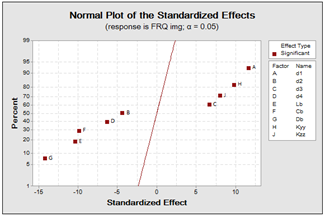Figure 6. Representation of the standardized effects as an imaginary part

The hydrodynamic bearings dimensions of Db, Lb, Cb and their linked interactions are all important (α = 0.05) (see Figure 7).

## 7.png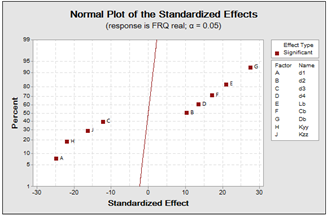Figure 7. Representation of the standardized effects as a real part

## 8.png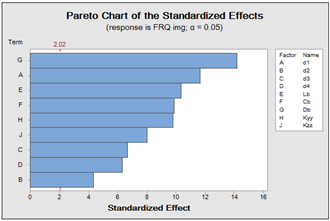Figure 8. Pareto chart of the standardized effects- imaginary part

We note that it is the factor that positively affects the frequency in the imaginary part has negatively affected the frequency in the real part.

The effects absolute values are displays by Minitab on the Pareto chart (see Figures 8, 9). All effects behind the reference line are significant at the level of 0.05, in the imaginary part. The diameter D1 and stiffness kyy are all important (α = 0.05), in the real part we find the opposite.Db and Lb all important (α = 0.05).

## 9.png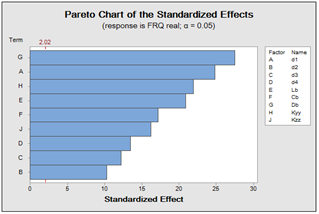Figure 9. Pareto chart of the standardized effects- real part

## 10.png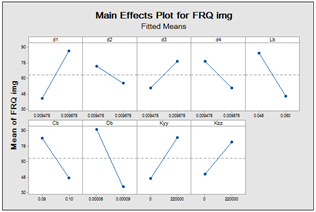Figure 10. Main effects plot for frequency- imaginary part

## 11.png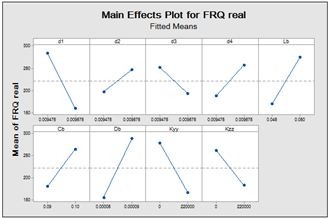Figure 11. Main effects plot for frequency- real part

Then, the principal effect plots are sketched in MINITAB 17 as illustrated in Figures 10 and 11. The different diameters effects, the stiffness, and the hydrodynamic bearing dimensions on the excitation frequency show:

• For imaginary part

The diameter d1 and the stiffness kzz, kyy have significant effect where they augment the excitation frequency. The plot also reports that:

• The diameter d1 has more influence on the frequency compared to the stiffness kzz, kyy.

• The other hydrodynamic bearing diameters and dimensions of (Lb, Db, and Cb( don’t have an important effect on the excitation frequency.

• For real part

The hydrodynamic bearing dimensions of (Db, Lb, and Cb ( and diameters of D4 and D2 have significant effect where they augment the excitation frequency. The plot also reports that:

• The hydrodynamic bearing dimensions of (Db, Lb, and Cb( have significant effect on the frequency compared to the diameters D4 and D2.

• The other diameters and the stiffness don’t have a significant effect on the excitation frequency.

An improvement chart gives the effect of each factor (columns) on responses (rows) (Figure 12). The vertical red lines on the graph represent the current operator settings. The numbers displayed above the column indicate the current factor level settings (red color). The blue horizontal lines and numbers represent responses to the current factor level.

Minitab calculates in the imaginary part the diametre d1 and kzz are manimized when all factors are at their highest settings (d1=0,0096, kzz=195555,556).

In real part the hydrodynamic bearing dimensions of (Db, Lb, and Cb (and diameters of D4 and D2, are manimized when all factors are at their Its lowest settings (Db=0.0001, Lb=0.0450, Cb= 0.090, D4= 0.0095, D2 =0.0095).

## 12.pngFigure 12. Optimum solution for nine factors

You like to see how the responses change when the stiffness kzz =166666.667. In interactive mode, you can move the factor level (red) line for stiffness kzz, or enter 166666.667 in the stiffness kzz.The graphic shows that the expected response in the imaginary part (86.0828) and the expected response in the real part (191.2315).

Pareto charts Figure 13 are a type of bar chart in which the horizontal axis represents attributes of interest, rather than a continuous scale. Typically, these features are "disadvantages". When arranging bars from largest to smallest, a Pareto chart can help you identify errors that are made up of a small number of vital elements and which are of little significance. The Cumulative Percentage line helps you determine the added contribution of each of the categories. Pareto Charts can also help focus improvement efforts on areas where the greatest gains can be made.

a- The imaginary part:

The vital few shortness in the imaginary part is represented by the following values of the imaginary frequency (Figure 13).

FRQ img =142.210, FRimg =142.60, FRQ img =144.570

FRQ img =144.900, FRimg =145.210, FRimg =145.560

## 13.png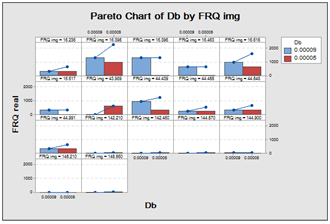Figure 13. Pareto charts " imaginary part"

b-The real part:

The vital few shortness in the real part is represented by the following values of the real frequency (Figure 14).

FRQ real =310.263, FR real =310.516. FR real =323.984.

FRQ real =324.052, FR real =324.409, FR real =325.855.

## 14.pngFigure 14. Pareto charts

After determining d1 as the main diameter affecting excitation frequency imaginary part, and the Db outer journal diameter as the main diameter affecting excitation frequency in real part, optimization charts are drawn based on values of frequencies obtained from matlab software. Figure 15 shows the model for rotor kit with various sections, disc and bearings. Figure 16 illustrates the Campbell diagram of the rotor-shaft system, where the shafts internal material damping is taken into consideration. The graph is plotted using the whirl frequencies (found from imaginary part of the eigenvalues), which are two positions, the first position in reverse rotation "BW", where the rotor rotates in the opposite direction. The second position is the rotation "FW", where the rotor rotates in the direction of rotation. The critical speed corresponding to the first position and the critical speed corresponding to the second position appear, the values of the first critical speeds identified.

## 15.png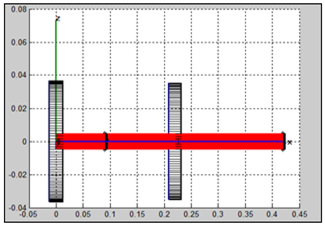Figure 15. Rotor kit with various sections

## 16.pngFigure 16. Campbell diagram

Critical speeds – Total number of modes studied 5.

Mode       (Hz)               (rpm)

3     2.6233e-002     1.5740e+000

4     2.3259e-001     1.3955e+001

5     4.5778e+001     2.7467e+003

Figure 17 presents the evolution of the damping constant as a function of the rotational speed. Through the diagram, we notice that the values of the damping factor are negative and thus indicate that the rotor is stable.

## 17.png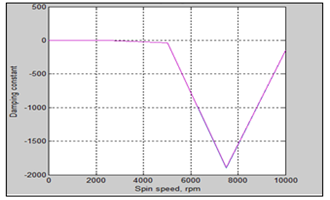Figure 17. Stability diagram

Onset of instability speeds

Mode       (Hz)                 (rpm)

1     2.8279e+001     1.6967e+003

2     9.8980e+001     5.9388e+003

3     0.0000e+000     0.0000e+000

4     0.0000e+000     0.0000e+000

5     0.0000e+000     0.0000e+000

Table 9 gives the result of the shapes of the modes and the shape precession and the rotational speed for the modes correspondent to critical speeds. The modes 3, 4, 5, are direct precession (the rotor rotates in the direction of rotation).

Figure 18 gives relative deviation as a function of tree length and confirms the results obtained in Table 9.

## 18.png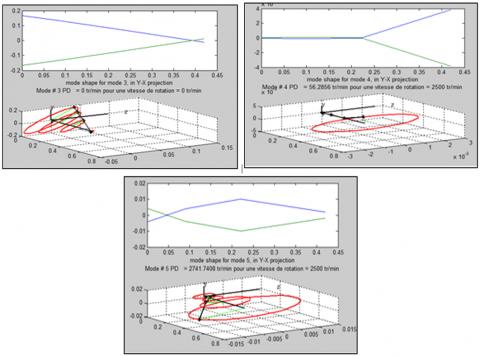Figure 18. Forms of modes and precession of forms of modes at 0 rpm

Table 9. Forms of modes and precession of forms at 0 rpm

 Modes Precession Spin speed rpm 3 direct 0 rpm 4 direct FB=56.2856 rpm 5 direct FB=2741.7408 rpm
8. Conclusions

The Plackett-Burman method in DOE and finite elements were employed to optimize the rotor and investigate the effects of the hydrodynamic bearings dimensions and the stiffness on the rotating machines dynamics and also to know the diameters which produce considerable effects on the excitation frequency as well as the reactions which can increase or decrease the principal effects and the gyroscopic effect on the eigenvalues of rotor-bearing systems. Our results show that:

-The diameter D1 has the more significant positive effect on the real part frequency which can be seen in the right of the response line compared to outer journal diameter Db that has a negative effect on the imaginary part frequency which is shown in the left of the response line and vice versa, as affirmed by the plot of principal effects.

-By the consideration of the internal material damping of the rotor. During the forward whirl, damping decreases, as the spin speed augments and in backward whirl, damping augments, as the spin speed augments. So, the system stability is determined. the stability of the system indicates the positive value of damping factor.

-For the system stability, it’s required to be operated at a speed lesser than a critical speed. From the maximum real part vs the spin speed plot, the stability can be determined. It’s concluded that the system is unstable for the positive value of the maximum real part, and it’s stable for the negative value of the real part. As conclusion, the modal analysis is a main tool to get an important idea about the system dynamic behavior.

Nomenclature
 Lb length of bearings [m]. Db outer journal diameter [m]. Cb bearing clearance [m]. Kyy, Kzz stiffness coefficients Cyy, Czz damping coefficients a gyroscopic effect k1, k2 stiffness ω The speed of rotation of the shaft (rd / s) Ω angular velocity (shaft) (rd / s) [K b] [C b] dimensionless stiffness coefficients. dimensionless damping coefficients. DF Degrees of freedom from each source SS Sum of squares. MS Mean squares. F Calculate by dividing the factor MS by error. P Use to determine whether a factor is signif. Secoff Standard error of the coefficient. S Estimated standard deviation of the error. Seq SS Sequential sum of squares. Adj SS Adjusted sum of squares. F The degrees of freedom for the test
References

 Childs, D. (1993). Turbomachinery Rotordynamics. John Wiley & Sons, Inc, New York.

 Vance, J.M. (1988). Rotordynamics of Turbomachinery. John Wiley & Sons, Inc, New York.

 Decaestecker, T.N., Lambert, W.E., Peteghem Carlos, H.V., Deforce, D., Van Bocxlaer, J.F. (2004). Optimization of solid-phase extraction for a liquid chromatographic-tandem mass spectrometric general unknown screening procedure by means of computational techniques. J Chrom A, 1056(1-2): 57-65. https://doi.org/10.1016/j.chroma.2004.06.010

 http://www.doesinc.com/knowledge.htm, accessed on Sep. 2006.

 Rao J.S. (1996). Rotor Dynamics. New Age International Publishers.

 Pilkey, W.D., Wang, B.P., Vannoy, D. (1976). Efficient optimal design of suspension systems for rotating shafts. J. Eng. Ind., 98(3): 1026-1029. https://doi.org/10.1115/1.3438996

 Bhat, R.B., Rao, J.S., Sankar, T.S. (1982). Optimum journal bearing parameters for minimum rotor unbalance response in synchronous whirl. J. Mech. Des., 104(2): 339-344. https://doi.org/10.1115/1.3256349

 Stocki, R., Szolc, T., Tauzowski, P. (2012). Robust design optimization of the vibrating rotor-shaft system subjected to selected dynamic constraints. Mech Syst Signal Process 2012; 29: 34-44. https://doi.org/10.1016/j.ymssp.2011.07.023

 Helfrich, R., Wagner, N. (2015). Application of optimization methods in rotor dynamics. In: Proceedings of the 9th IFToMM International Conference on Rotor Dynamics, pp. 1715-1725. https://doi.org/10.1007/978-3-319-06590-8_141

 Yücel, E., Saruhan, H. (2017). Design optimization of rotor-bearing system considering critical speed using Taguchi method. Proc IMechE, Part E: J Process Mechanical Engineering, 231(2): 138-146. https://doi.org/10.1177/0954408915578581

 Jeffcott, H. (1919). The lateral vibration of loaded shaft in neighbourhood of a whirling speed - the effect of want of balance. Phil. Mag., 37: 304-314. https://doi.org/10.1080/14786440308635889

 Irretier, H. (1999). Mathematical foundations of experimental modal analysis in rotor dynamics. Mechanical Systems and Signal Processing, 13(2): 183-191. https://doi.org/10.1006/mssp.1998.1215

 Rao J.S. (1996). Rotor Dynamics. New Age International Publishers.

 Choses, M., Dutt, J.K., Modak, S.V. (2012). Modal analysis of rotor-shaft system under the influence of rotor-shaft material damping and fluid film forces. Mechanism and Machine Theory, 48: 81-93. https://doi.org/10.1016/j.mechmachtheory.2011.09.001

 Forrai, L. (2000). A finite element model for stability analysis of symmetrical rotor systems with internal damping. Journal of Computational and Applied Mechanics, 1(1): 37-47.

 Fegade, R., Patel, V. (2013). Unbalanced response and design optimization of rotor by Ansys and design of experiments. International Journal of Scientific &   Engineering Research, 4(7).

 Fegade, R., Patel, V., Nehete, R.S., Bhandarkar, B.M. (2014). Unbalanced response of rotor using ansys parametric design for different bearings. International Journal of Engineering Sciences & Emerging Technologies, 7(1): 506-515.

 Mostefa, B., Kaddour, R., Mimoun, Y., Abdallah, N. (2018). Plackett-burman desing to study the influence of the stiffness of hydrodynamic bearings on the dynamic behaviour of turbo machinery. Mathematical Modelling of Engineering Problems, 5(4): 407-417. https://doi.org/10.18280/mmep.050418

 Naouri Abdallah Mostefa, B., Kaddour, R., Mimoun, Y. (2020). Investigate the effect of damping parameters of the hydrodynamic bearings using the optimization method of design of experiments. Mathematical Modelling of Engineering Problems, 7(1): 103-112. https://doi.org/10.18280/mmep.070113

 Someya, T. (ed). (1988). Journal-Bearing Databook. Springer, Berlin. https://doi.org/10.1007/978-3-642-52509-4

 Atherton, D.P. (1975). Nonlinear Control Engineering. Van Nostrand Reinhold Company.

 Meirovitch, L. (1986). Elements of Vibration Analysis. Mac Graw Hill International Editions.

 Sino, R. (2007). Comportement dynamique et stabilité des rotor: application aux rotors composites. Thèse doctorat INSA Lyon, P187.

 Dugundji, J., Wendelle. J.H. (1983). Some analysis methodes for rotating systems with periodic coefficient. American Institute of aeronautics journal, 21(6): 890-897. https://doi.org/10.2514/3.8167

 Meng, G., Gasch, R. (2000). Stability and stabillity degree of a cracked flexible rotor supported on journal bearing. Journal of Vibration and Acoustics, 122: 116-125. https://doi.org/10.1115/1.568448